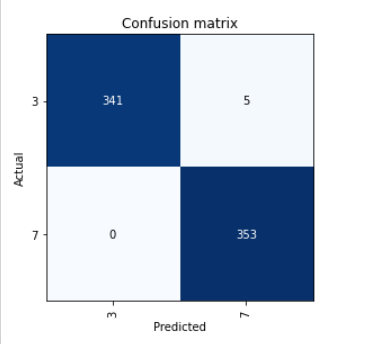# Unable to plot confusion matrix

I have a large dataset of 194082 images and I have trained a model on it. I am unable to plot the confusion matrix for the same. The kernel runs out of memory and dies. This is the case on Kaggle as well as Paperspace (free account). Is there a memory-efficient way to plot the matrix?
Thanks

Out of curiosity, could you include the out of memory error? Just wanting to see where it is dying. I wouldn’t see why confusion matrix would take much memory.

1 Like

This doesn’t fix your plot_confusion_matrix problem, but .most_confused() gives the same information in a list format. You might try .most_confused(5) to get the top 5 wrong predictions.

1 Like

Thank you for the reply however I really need the confusion matrix or the false negatives to be precise since those are what I’m trying to minimize

Thank you for the response. It’s not really an error I can copy. On Kaggle the page just refreshes with a red bar called “Tried to allocate more memory than available, kernel died.” On paper space as well the kernel just dies so I assume it’s the same error.

Oh, I just checked the documentation.
“.confusion_matrix()” returns an matrix with the information.

I tried to do the following:

``````vpreds = learn.get_preds(1) #get preds for validation set
y_preds = list(map(int, vpreds)) #turn them into a list of ints
y_true = list(dls.valid_ds.items['any']) # get the validation set ground truths that are stored in a df column called any
assert len(y_true) == len(y_preds)
``````

After this I am doing

``````from sklearn.metrics import confusion_matrix
tn, fp, fn, tp = confusion_matrix(y_true, y_preds).ravel()
fp, fn
``````

and the output is

``````0, 0
``````

This does not make sense because when I train the model, I print accuracy and its about ~89%. Also when I plot `learn.show_results()` it shows some wrong predictions.

What am I doing wrong?

Also `learn.get_preds` has two attributes save preds and save targs but I’m not able to understand how to use them.

see if this works which I used in Visual GUI but for v1.

interp = ClassificationInterpretation.from_learner(learn)

upp, low = interp.confusion_matrix()
tn, fp = upp, upp
fn, tp = low, low
print(tn, fp, fn, tp)

Hey, thank you for the response.

This line of code only fails. I am not able to do anything further

hmmm… here is the test code I am using:

source = untar_data(URLs.MNIST_TINY)
items = get_image_files(source)

item_tfms=RandomResizedCrop(128, min_scale=0.35), batch_tfms=Normalize.from_stats(*imagenet_stats))

dblock = DataBlock(blocks = (ImageBlock, CategoryBlock),
get_items = get_image_files,
get_y = parent_label,
splitter = GrandparentSplitter(),
item_tfms = RandomResizedCrop(128, min_scale=0.35),
batch_tfms=Normalize.from_stats(*imagenet_stats))

learn = cnn_learner(dls, resnet34, metrics=accuracy, pretrained=True)
learn.fit_one_cycle(3, 1e-2)

interp = ClassificationInterpretation.from_learner(learn)
losses,idxs = interp.top_losses()
len(dls.valid_ds)==len(losses)==len(idxs)
interp.plot_confusion_matrix()

This is the confusion matrixupp, low = interp.confusion_matrix()
tn, fp = upp, upp
fn, tp = low, low
print(tn, fp, fn, tp)

results in

341 5 0 353

I should have added all the linesinterp = ClassificationInterpretation.from_learner(learn)
losses,idxs = interp.top_losses()
len(dls.valid_ds)==len(losses)==len(idxs)
interp.plot_confusion_matrix()

Hey, thanks again. It works with me as well for smaller datasets. Only when the dataset is large it gives out of memory error. What platform are you using btw?

Using windows, not sure about the issues with large datasets